Related Articles
Make subplots span multiple grid rows and columns in Matplotlib
• Last Updated : 26 Dec, 2020

In this article, we are going to discuss how to make subplots span multiple grid rows and columns using matplotlib module.

For Representation in Python, matplotlib library has been the workhorse for a long while now. It has held its own even after more agile opponents with simpler code interface and abilities like seaborn, plotly, bokeh and so on have shown up on the scene. Despite the fact that Matplotlib may come up short on the intuitive capacities of the tenderfoots, it does an above and beyond the employment of imagining our information investigation undertakings in Exploratory Information Analysis(EDA).

During EDA, one may run over circumstances where we need to show a gathering of related plots as a component of a bigger picture to commute home our knowledge. The subplot capacity of matplotlib takes care of the work for us. Be that as it may, in specific circumstances, we might need to join a few subplots and need to have distinctive angle proportions for each subplot.

Firstly, import gridspec submodule of matplotlib module.

## Python3

 `# import modules``import` `matplotlib.pyplot as plt``from` `matplotlib.gridspec ``import` `GridSpec`` ` `# create objects``fig ``=` `plt.figure()``gs ``=` `GridSpec(``4``, ``4``, figure``=``fig)`

We first need to make an object of GridSpec which permits us to indicate the absolute number of lines and segments as contentions in the general figure alongside a figure object.

We store the GridSpec object in a variable called gs and indicate that we need to have 4 lines and 4 segments in the general figure.

Below are some programs to make subplots span multiple grid rows and columns:

Example 1:

## Python3

 `# explictit function to hide index ``# of subplots in the figure``def` `formatAxes(fig):``    ``for` `i, ax ``in` `enumerate``(fig.axes):``        ``ax.text(``0.5``, ``0.5``, ``"ax%d"` `%` `(i``+``1``), va``=``"center"``, ha``=``"center"``)``        ``ax.tick_params(labelbottom``=``False``, labelleft``=``False``)`` ` ` ` `# import required modules``import` `matplotlib.pyplot as plt``from` `matplotlib.gridspec ``import` `GridSpec`` ` `# create objects``fig ``=` `plt.figure(constrained_layout``=``True``)``gs ``=` `GridSpec(``3``, ``3``, figure``=``fig)`` ` `# create sub plots as grid``ax1 ``=` `fig.add_subplot(gs[``0``, :])``ax2 ``=` `fig.add_subplot(gs[``1``, :``-``1``])``ax3 ``=` `fig.add_subplot(gs[``1``, : ``-``1``])``ax4 ``=` `fig.add_subplot(gs[``-``1``, ``0``])``ax5 ``=` `fig.add_subplot(gs[``-``1``, ``-``2``])`` ` `# depict illustartion``fig.suptitle(``"Grid-Spec"``)``formatAxes(fig)``plt.show()`

Output: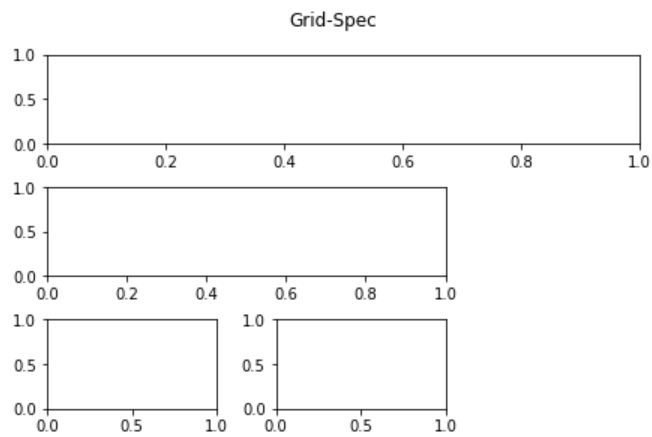Presently, we need to determine the subtleties of how each subplot will traverse the lines and segments in the general figure. It is valuable to make a harsh sketch on paper regarding how you need the subplots to be spread out, so they don’t cover. When done, we pass on this data through the GridSpec object we made. The line/segment length information is passed in similar list documentation we use for subsetting exhibits/dataframes with lines and segment list numbers beginning from zero and utilizing the ‘:’ to indicate the range. The GridSpec object with the file is passed to the add_subplot capacity of the figure object.

We add a general title for the figure and eliminate the ticks to envision the format better as the goal here is to exhibit how we can accomplish subplots spreading over different lines/segments. At the point when you actualize this, clearly, you will need to add your pivot ticks, names and so forth from your dataframe and change the dividing and figure size to oblige these plot components.

Example 2:

Here, we have created an explicit function to format axes of the figure i.e. hide the index values of the sub plots.

## Python3

 `# explictit function to hide index ``# of sub plots in the figure``def` `formatAxes(fig):``    ``for` `i, ax ``in` `enumerate``(fig.axes):``        ``ax.text(``0.5``, ``0.5``, ``"ax%d"` `%` `(i``+``1``), va``=``"center"``, ha``=``"center"``)``        ``ax.tick_params(labelbottom``=``False``, labelleft``=``False``)`` ` `# import required modules``import` `matplotlib.pyplot as plt``from` `matplotlib.gridspec ``import` `GridSpec`` ` `# create objects``fig ``=` `plt.figure(constrained_layout``=``True``)``gs ``=` `GridSpec(``3``, ``3``, figure``=``fig)`` ` `# create sub plots as grid``ax1 ``=` `fig.add_subplot(gs[``0``, :])``ax2 ``=` `fig.add_subplot(gs[``1``, :``-``1``])``ax3 ``=` `fig.add_subplot(gs[``1``:, ``-``1``])``ax4 ``=` `fig.add_subplot(gs[``-``1``, ``0``])``ax5 ``=` `fig.add_subplot(gs[``-``1``, ``-``2``])`` ` `# depict illustration``fig.suptitle(``"GridSpec"``)``formatAxes(fig)``plt.show()`

Output: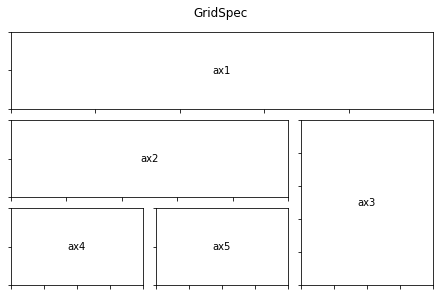This may prove to be useful in multi-variable time arrangement plots where we might need to show the time arrangement plot extending across the sections in the top column and other uni-variate, multi-variate perception in the other subplots underneath. You can tweak how your jigsaw looks like by indicating your line/sections in the general figure and ranges of your individual subplots.

Example 3:

Combining two subplots utilizing subplots and GridSpec, here we need to consolidate two subplots in a tomahawks format made with subplots. We can get the GridSpec from the tomahawks and afterward eliminate the covered tomahawks and fill the hole with another greater tomahawks. Here we make a format with the last two tomahawks in the last section joined.

See additionally Modifying Figure Formats Utilizing GridSpec and Different Capacities.

## Python3

 `# import required modules``import` `matplotlib.pyplot as plt``from` `matplotlib.gridspec ``import` `GridSpec`` ` `# create objects``fig, axes ``=` `plt.subplots(ncols``=``3``, nrows``=``3``,)``gs ``=` `axes[``1``, ``2``].get_gridspec()`` ` `# create sub plots as grid``for` `ax ``in` `axes[``1``:, ``-``1``]:``    ``ax.remove()``axsbig ``=` `fig.add_subplot(gs[``1``:, ``-``1``])``axsbig.annotate(``'Big Axes \nGridSpec[1:, -1]'``,``                ``(``0.1``, ``0.5``),``                ``xycoords``=``'axes fraction'``,``                ``va``=``'center'``, color``=``"g"``)`` ` `# depict illustartion``fig.tight_layout()``plt.show()`

Output: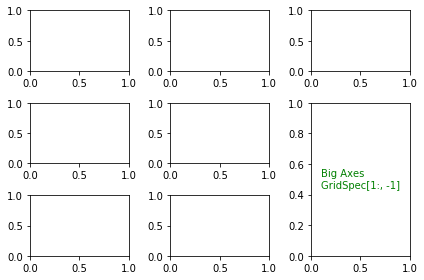Example 4:

In this example, we are going to add a subplot that spans two rows.

## Python3

 `# import required modules``import` `matplotlib.pyplot as plt``from` `matplotlib.gridspec ``import` `GridSpec`` ` `# create objects``fig ``=` `plt.figure()``gridspan ``=` `fig.add_gridspec(``2``, ``2``)`` ` `# create sub plots as grid``ax1 ``=` `fig.add_subplot(gs[``0``, ``0``])``ax2 ``=` `fig.add_subplot(gs[``1``, ``0``])``ax3 ``=` `fig.add_subplot(gs[:, ``-``1``])`` ` `# depict illustartion``plt.show()`

Output: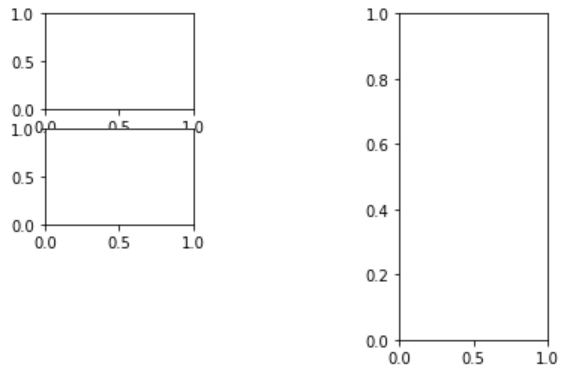Example 5:

Here, we illustrate an application of subplots by depicting various graphs in different grids of the illustration.

## Python3

 `# Import libraries``import` `numpy as np``import` `matplotlib.pyplot as plt`` ` `# Create a 2x2 grid of plots``fig, axes ``=` `plt.subplots(``2``, ``2``, constrained_layout``=``True``)``a ``=` `np.linspace(``0``, ``1``)`` ` `# Modify top-left plot``axes[``0``,``0``].set_title(``"Top--Left"``, color``=``"g"``)``axes[``0``,``0``].plot(x, x)`` ` `# Modify top-right plot``axes[``0``,``1``].set_title(``"Top--Right"``, color``=``"r"``)``axes[``0``,``1``].plot(x, x``*``*``2``)`` ` `# Modify bottom-left plot``axes[``1``,``0``].set_title(``"Bottom--Left"``, color``=``"b"``)``axes[``1``,``0``].plot(x, np.sin(``3``*``x))`` ` `# Modify bottom-right plot``axes[``1``,``1``].set_title(``"Bottom--Right"``)``axes[``1``,``1``].plot(x, ``1``/``(``1``+``x))`` ` `# Depict illustration``plt.show()`

Output: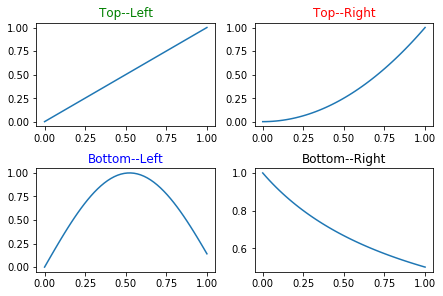Attention geek! Strengthen your foundations with the Python Programming Foundation Course and learn the basics.

To begin with, your interview preparations Enhance your Data Structures concepts with the Python DS Course.

My Personal Notes arrow_drop_up